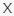# Video Math Quiz VideoQuizStar 2

Q 1. Let’s start with an easy one. Click PLAY to watch the video below and see if you can answer the question.

Choices:

A – 8%
B – 20%
C – 25%
D – 33%

B – 20%

Q 2. Watch the video below and try to answer this question about geometry! (Have a look at this triangle!)

Choices:

5
6
7
12

5

Q 3. Pay close attention to the video below. Can you answer the question? (How many spheres were there?)

Choices:

A – 156
B – 158
C – 161
D – 164
E – 166
F – 169

C – 161

Try Version1: Video Math Quiz VideoQuizStar

Q 4. Don’t lose focus on this one. Can you solve the equation in the video? (Solve the equation)

Choices:

A – 3
B – 8
C – 12
D – 18

B – 8

Try Version1: Video Math Quiz VideoQuizStar

Q 5. Don’t lose focus on this one. Can you solve the equation in the video? (Solve the equation)

Choices:

A – 0.0625
B – 0.25
C – 13
D – 17.5
E – 52
F – 70
G – 94
H – 367

E – 52

Q 6. Let’s test your logic skills! Watch the video and try to find the correct answer! (Determine the value of each object to solve the equation)

Choices:

A
B
C
D
E
F